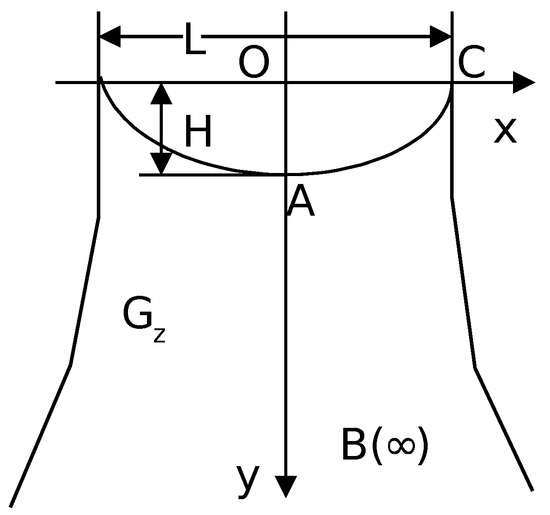Next Article in Journal
Approximate Controllability of Semilinear Stochastic Integrodifferential System with Nonlocal Conditions
Next Article in Special Issue
Regularized Integral Representations of the Reciprocal Gamma Function
Previous Article in Journal
Fatigue-Induced Cole Electrical Impedance Model Changes of Biceps Tissue Bioimpedance
Previous Article in Special Issue
Power Laws in Fractionally Electronic Elements
Open AccessArticle

Mathematical Modeling of Solutes Migration under the Conditions of Groundwater Filtration by the Model with the k-Caputo Fractional Derivative

VM Glushkov Institute of Cybernetics of NAS of Ukraine, Kyiv 03680, Ukraine
*
Author to whom correspondence should be addressed.
Fractal Fract 2018, 2(4), 28; https://doi.org/10.3390/fractalfract2040028
Received: 1 October 2018 / Revised: 20 October 2018 / Accepted: 23 October 2018 / Published: 24 October 2018
(This article belongs to the Special Issue The Craft of Fractional Modelling in Science and Engineering 2018)
Within the framework of a new mathematical model of convective diffusion with the k-Caputo derivative, we simulate the dynamics of anomalous soluble substances migration under the conditions of two-dimensional steady-state plane-vertical filtration with a free surface. As a corresponding filtration scheme, we consider the scheme for the spread of pollution from rivers, canals, or storages of industrial wastes. On the base of a locally one-dimensional finite-difference scheme, we develop a numerical method for obtaining solutions of boundary value problem for fractional differential equation with k-Caputo derivative with respect to the time variable that describes the convective diffusion of salt solution. The results of numerical experiments on modeling the dynamics of the considered process are presented. The results that show an existence of a time lag in the process of diffusion field formation are presented. View Full-Text
Show FiguresFigure 1

MDPI and ACS Style

Bohaienko, V.; Bulavatsky, V. Mathematical Modeling of Solutes Migration under the Conditions of Groundwater Filtration by the Model with the k-Caputo Fractional Derivative. Fractal Fract 2018, 2, 28.

Show more citation formats Show less citations formats
Note that from the first issue of 2016, MDPI journals use article numbers instead of page numbers. See further details here.

1